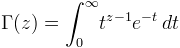Computes the Gamma function.

Namespace:  Meta.Numerics.Functions
Assembly:  Meta.Numerics (in Meta.Numerics.dll) Version: 4.1.4Syntax
```public static double Gamma(
double x
)```

#### Parameters

x
Type: SystemDouble
The argument.

#### Return Value

Type: Double
The value of Γ(x).Remarks

The Gamma function is a generalization of the factorial (see Factorial(Int32)) to arbitrary real values.For positive integer arguments, this integral evaluates to Γ(n+1)=n!, but it can also be evaluated for non-integer z.

Like the factorial, Γ(x) grows rapidly with increasing x; Γ(x) overflows Double for all x larger than ~171. For arguments in this range, you may find it useful to work with the LogGamma(Double) method, which returns accurate values for ln(Γ(x)) even in the range for which Γ(x) overflows.

To evaluate the Gamma function for a complex argument, use Gamma(Complex).

## Domain, Range, and Accuracy

The function is defined for all x. It has poles at all negative integers and at zero; the method returns NaN for these arguments. For positive arguments, the value of the function increases rapidly with increasing argument. For values of x greater than about 170, the value of the function exceeds MaxValue; for these arguments the method returns PositiveInfinity. The method is accurate to full precision over its entire domain.See Also